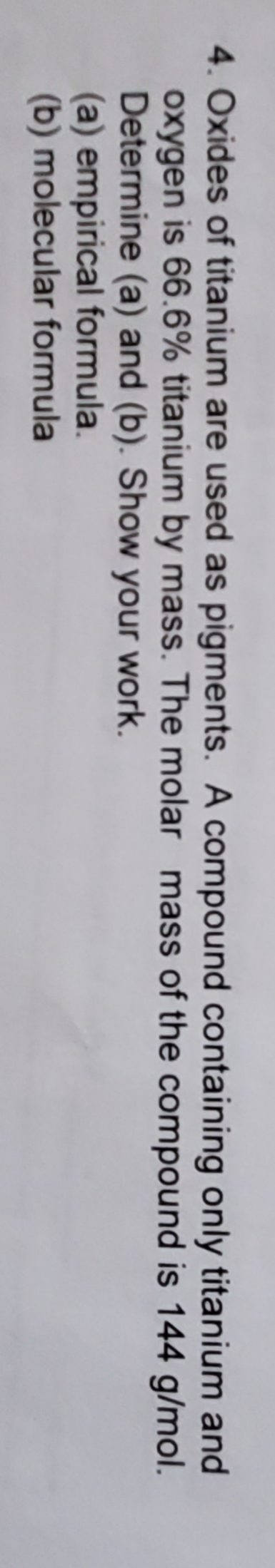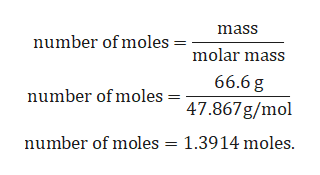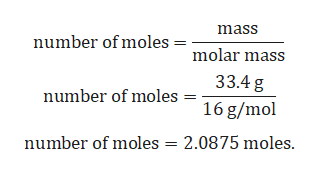# 4. Oxides of titanium are used as pigments. A compound containing only titanium andoxygen is 66.6% titanium by mass. The molar mass of the compound is 144 g/mol.Determine (a) and (b). Show your work(a) empirical formula(b) molecular formula

Question
3 viewshelp_outlineImage Transcriptionclose4. Oxides of titanium are used as pigments. A compound containing only titanium and oxygen is 66.6% titanium by mass. The molar mass of the compound is 144 g/mol. Determine (a) and (b). Show your work (a) empirical formula (b) molecular formula fullscreen
check_circle

Step 1

Given:

Compound contains only titanium and oxygen.

Percent mass of titanium = 66.6 %.

Percent mass of oxygen = 100% - 66.6% = 33.4 %.

Molar mass of oxygen = 16 g/mol.

Molar mass of titanium = 47.867 g/mol.

Molar mass of compound = 144 g/mol.

Conversion of mass percent to gram:

Since we are having mass percent, it is convenient to use 100 g of the compound as the starting material. Thus, in the 100 g of sample of above compound 66.6 g of titanium and 33.4 g of oxygen.

Step 2

Calculation for number of titanium:help_outlineImage Transcriptionclosemass number of moles molar mass 66.6 g number of moles 47.867 g/mol number of moles = 1.3914 moles fullscreen
Step 3

Calculation for numb...help_outlineImage Transcriptionclosemass number of moles molar mass 33.4 g number of moles 16 g/mol number of moles 2.0875 moles fullscreen

### Want to see the full answer?

See Solution

#### Want to see this answer and more?

Solutions are written by subject experts who are available 24/7. Questions are typically answered within 1 hour.*

See Solution
*Response times may vary by subject and question.
Tagged in

### Chemical bonding